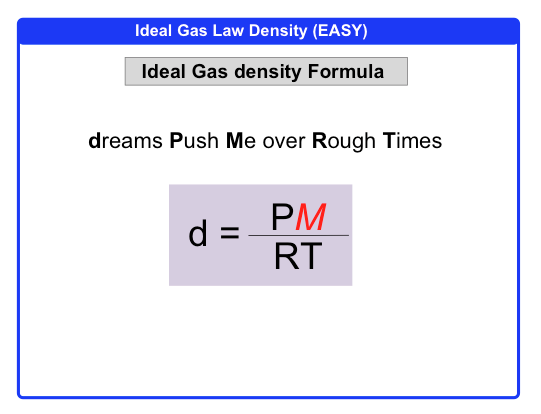Clutch Prep is now a part of Pearson

# The Ideal Gas Law: Density

See all sections
Sections
Pressure Units
The Ideal Gas Law
The Ideal Gas Law Derivations
The Ideal Gas Law Applications
Chemistry Gas Laws
Chemistry Gas Laws: Combined Gas Law
Mole Fraction
Partial Pressure
The Ideal Gas Law: Molar Mass
The Ideal Gas Law: Density
Gas Stoichiometry
Standard Temperature and Pressure
Effusion
Root Mean Square Speed
Kinetic Energy of Gases
Maxwell-Boltzmann Distribution
Velocity Distributions
Kinetic Molecular Theory
Van der Waals Equation
Boyle's Law (IGNORE)
Charles Law (IGNORE)
Ideal Gas Law (IGNORE)

Under certain conditions of pressure and temperature, the Ideal Gas Law can determine the density of a gas.

###### The Ideal Gas Law (Density)

Concept #1: Density

Example #1: An unknown gas sample has a density of 1.70 g/L. If the sample has a volume 120.0 mL, what is its mass in grams?

Concept #2: Ideal Gas Law Density (EASY)Concept #3: Ideal Gas Law Density (HARD)

Example #2: A gaseous compound of nitrogen and hydrogen is found to have a density of 0.977 g/L at 0.69474 atm and 373.15 K. What is the molecular formula of the compound?

Practice: Consider two containers of gases at the same temperature. One has helium at a pressure of 1.00 atm. The other contains carbon dioxide with the same density as the helium gas. What is the pressure of the carbon dioxide gas sample?

Practice: Determine the molecular formula of a gaseous compound that is 49.48% carbon, 5.19% hydrogen, 28.85% nitrogen, and 16.48% oxygen. At 27°C, the density of the gas is 1.5535 g/L and it exerts a pressure of 0.092 atm.